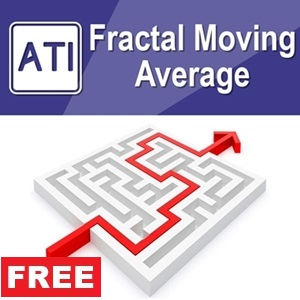•### Introduction to Fractal Moving Average Indicator

Fractal Moving Average and Fractal Pattern Indicator

Fractal Moving Average indicator was designed as part of Fractal Pattern Indicator. Inside Fractal Pattern Indicator, Fractal Moving Average Indicator was implemented as multiple of chart objects. With over thousands of chart object, we find that it was not the best and efficient ways to operate the indicator. It was computationally too heavy for the indicator. Hence, we decide to implement the indicator version of Fractal Moving Average indicator to benefit our Fractal Pattern Indicator user. Since this is free indicator, anyone can use. However, since there is some algorithm link between Fractal Moving Average and Fractal pattern Indicator, free users can not access some of its features like signal alerts.

How to use Fractal Moving Average Indicator

Fractal Moving Average Indicator is the great moving average that helps you to detect the market momentum. Especially, it draws the rectangle signal in your chart when moving average cross over or moving average cross down happens. The best practice is to catch momentum after the turning point is identified. Hence, you can get the long profitable range by connecting turning point with trend. Turning point identification can be done with Fractal pattern Indicator.

Input setting

Fractal Moving Average Indicator has only four inputs. It is simple to use.

• Bars To Scan: Number of bars to be included in calculation
• Fractal Moving Average Period: This is the indicator period for calculation
• Color of Cross Over: this is color of Rectangle when moving average cross over is happening
• Color of Cross Down: This is color of Rectangle signal when moving average cross down is happening
• Wick Line Width: thickness of Wick line on Cross Over (or Down) candle
• Candle Body Line Width: thickness of Body line on Cross Over (or Down) Candle
• Draw Arrow On Cross: You can choose to draw circle arrow on Cross Over (or Down) Candle

Basically, Fractal Moving Average indicator works best to catch moving average cross over and moving average cross down. Rectangles are drawn either when price is crossing over the Fractal Moving Average or when price is crossing down the Fractal Moving Average. Since some of its algorithm are connected to Fractal pattern Indicator, for sound, email and push notification to work, you need to have Fractal Pattern Indicator in the same chart. Basically, Fractal Moving Average barrows its alerts features from Fractal pattern Indicator. Sorry for the this limited alert feature but Fractal Pattern Indicator is highly sophisticated code with tons of code lines.

Important Note Before using Fractal Moving Average Indicator (Must read before using this indicator)

Fractal Moving Average indicator is completely free of charge. However, since it uses some algorithm from Fractal Pattern indicator, Fractal Moving Average indicator might come with some limitation for free users. Only use Fractal Moving Average indicator if you understand this limitation. We never push you to use this indicator. If you are already using Fractal Pattern Indicator, just ignore this warning.

You can get Fractal Pattern Indicator from link below:

Further Recommended Indicators for Fractal Moving Average Indicator

Below is the list of Recommended Tools for Fractal Moving Average Indicator.

Published Current Version Updated Visits
12 Feb 2020 2.3 28 Feb 2020 964

### More Products

PriceFreePaidPaid
Just enter 00000 to get going
in the Order ID field in